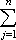#Interactive Real Analysis

Next | Previous | Glossary | Map

## 7.1. Riemann Integral

### Examples 7.1.11(a): Riemann Lemma

Is the function f(x) = x2 Riemann integrable on the interval [0,1] ? If so, find the value of the Riemann integral. Do the same for the interval [-1, 1] (since this is the same example as before, using Riemann's Lemma will hopefully simplify the solution).
When we proved before that the integral of f(x) = x2 exists and is equal to 1/3 we showed that
(*) U(f, P) - L(f, P)for every partition P with small enough norm. Using Riemann's Lemma it is enough to find one partition such that inequality (*) holds. Therefore, take the partition
P = {j/n, j = 0, 1, 2, ..., n}
for the interval [0, 1]. On every subinterval [(j-1)/n, j/n] the maximum of f occurs on the right, the minimum on the left. Therefore:
| U(f, P) - L(f, P) || f(j/n) - f((j-1)/n)| |1/n|
=| (j/n)2 - ((j-1)/n)2| |1/n| = 1 / n3| j2 - (j-1)2 |
= 1 / n3| j2 - j2 + 2 j - 1|2 / n3j
= 2 / n3 1/2 n (n+1) = (n+1) / n2
But since the last expression converges to zero as n goes to infinity, Riemann's Lemma shows that f(x) = x2 is indeed integrable over the interval [0, 1].

The remainder of this example is just as before (exercise!).

So, Riemann's lemma has indeed simplified our computation, because we are now able to pick one partition that is best suited for our particular function and/or interval.

Next | Previous | Glossary | Map